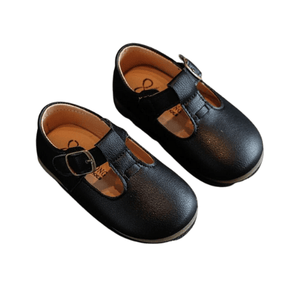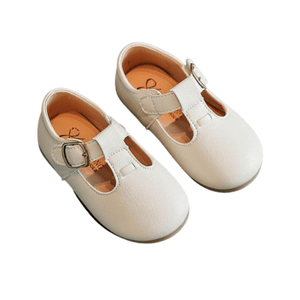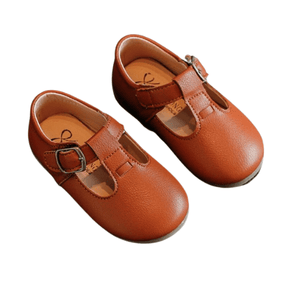# Brielle

Regular price 770.00
Tax included.

More toddler shoes selection!

Label Size = Size in CM = Size in Inches
21 = 13.7 = 5.39 inch
22 = 14.2 = 5.59 inch
23 = 14.7 = 5.79 inch
24 = 15.2 = 5.98 inch
25 = 15.7 = 6.18 inch
26 = 16.5 = 6.50 inch
27 = 17.1 = 6.73 inch
28 = 17.6 = 6.93 inch
29 = 18.2 = 7.17 inch
30 = 18.8 = 7.40 inch

x